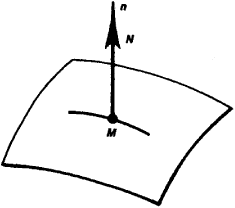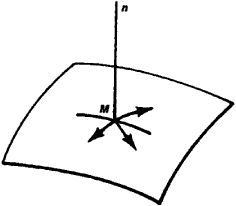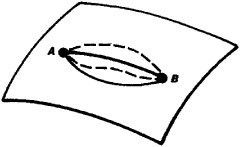# Variational Principles of Mechanics

The following article is from The Great Soviet Encyclopedia (1979). It might be outdated or ideologically biased.

## Variational Principles of Mechanics

The principles of mechanics are called those prime postulates that reflect equally general regularities of mechanical phenomena from which can be derived all the equations determining the motion of a mechanical system (or its equilibrium conditions). As mechanics developed, a number of such principles were established, each of which can form the basis of mechanics. This is explained by the variety of properties and laws of mechanical phenomena. These principles are divided into nonvariational and variational.

Nonvariational principles of mechanics directly establish the laws of motion performed by a system under the influence of the forces applied to it. Among these principles are Newton’s second law, according to which, in the motion of any point of a system, the product of its mass and its acceleration is equal to the sum of all the forces applied to it, and d’Alembert’s principle. The nonvariational principles hold true for any mechanical system and have a comparatively simple mathematical form. However, their application is limited only by the framework of mechanics, since such a purely mechanical concept as force enters into the expression of these principles. The following is also significant. In most mechanics problems, the motion of constrained systems, that is, systems whose motions are restricted by constraints, is considered. Examples of such systems are all kinds of machines and mechanisms, as well as land transport, where the constraints are bearings, hinges, cables, and the like and for land transport, road beds or rails as well. In order to study the motion of a system under constraint starting from nonvariational principles, it is necessary to replace the effect of the constraints by some force, called reaction (or also constraint). However, the magnitudes of these reactions are not known beforehand, since they depend on the magnitude and the point of application of the given (active) forces applied to the system—for example, the forces of gravity, elasticity of springs, thrust, and others—and likewise how the system itself moves under these conditions. Consequently, additional unknown quantities in the form of reactions are included in the constructed equations of motion, which usually considerably complicates the whole solution process.

The advantage of variational principles of mechanics is that equations of motion of the corresponding mechanical system that does not contain unknown reactions can be obtained directly from them. This is achieved because the effect of the constraints is accounted for not by their replacement by unknown forces (reactions) but by consideration of those motions or displacements (or velocity and acceleration increments) that the points of this system can have under the given constraints. For example, it point M moves along a given smooth (ideal) surface, which is a constraint for it (Figure 1), then the action of this constraint can be accounted for by replacing the constraint beforehand by a reaction N of previously unknown value, which is directed at any moment of time along the normal Mn toward the surface (since along this direction the constraint does not allow the point to move). But the effect of this same constraint can be accounted for by establishing that for a point in the given case at any position, only elementary displacements perpendicular to the normal Mn (Figure 2) are possible; such displacements are called virtual displacements. Finally, the effect of this same constraint can also be characterized by the fact that in this situation, the point’s motion from a certain position A to position B is possible only along a curve that lies on a surface that is a constraint (Figure 3); such motions are said to be kinematically realizable.Figure 1

The substance of the variational principles of mechanics is that they establish characteristics (indications) that make it possible to distinguish the true motion of a mechanical system, that is, motion actually occurring under the influence of specified forces, from one or another of its kinematically realizable motions (or the system’s equilibrium state from its other possible states). Usually, these characteristics (indications) consist of the fact that for a true motion some physical quantity that depends on the system’s characteristics has the smallest value in comparison with itsFigure 2

values over all the considered kinematically realizable motions. In this, the variational principles of mechanics can be distinguished from one another by the form of an indicated physical quantity and by the special features of the kinematically realizable motions under consideration, as well as by the special features of the mechanical systems themselves, for which these variational principles hold true. The use of variational principles requires the application of the methods of the calculus of variations.

According to their form, the variational principles of mechanics are divided into the so-called differential variational principles, in which it is established how the true motion of a system differs from the other kinematically realizable motions at each given moment of time, and the integral variational principles, in which this distinction is established for displacements performed by the system within some finite time interval.Figure 3

The differential variational principles of mechanics are, within the framework of mechanics, more general and are practically valid for any mechanical system. The integral variational principles of mechanics, in their most generally used form, hold true only for so-called conservative systems, that is, for systems in which the law of the conservation of mechanical energy is valid. However, these principles, in contrast to the differential variational principles and the nonvariational principles, in place of forces, deal with such physical quantities as energy, which permits the extension of these principles to nonmechanical phenomena, making them important in all of theoretical physics.

Among the fundamental differential variational principles of mechanics are the following: (1) The principle of virtual displacements, which establishes the equilibrium condition of a mechanical system under ideal constraints. According to this principle, the equilibrium position of a mechanical system differs from all its other possible positions in the equality to zero for equilibrium positions of the sum of the work elements of all the active forces applied to the system for any possible displacement of the system. (2) The d’Alembert-Lagrange principle, according to which a true motion of a mechanical system under ideal constraints differs from all kinematically possible motions in that only for a true motion, the sum at each moment of time of the work elements of all the active forces and all the inertial forces applied to the system for any possible displacement of the system is equal to zero. In these variational principles, the physical quantity under consideration is the work done by the forces.

Gauss’ principle (principle of “least constraint”) is also a differential variational principle of mechanics; for Gauss’ principle, the physical quantity under consideration is the so-called “constraint,” expressed in terms of the specified forces and the accelerations of the system’s points. There is also the Hertz principle (the principle of least curvature), which is closely related to Gauss’ principle.

Among the integral variational principles of mechanics are the principles of least (stationary) action. According to these principles the true motion, among those kinematically possible motions of a system between two of its positions, is the one for which the physical quantity, called the action, has a minimum value. Different forms of these variational principles are characterized by the choice of the quantity of action and by the special features of the kinematically possible motions being compared.

Both nonvariational and variational principles were established in the process of investigating the properties of mechanical systems and their laws of motion. Since mechanical phenomena, like other physical phenomena, obey many laws, the corresponding mechanical systems have been found to be governed by a number of principles, among them the variational principles. If any one of these principles is taken as the initial principle, then one can derive from it not only the equations of motion of a given system but also all the other principles that are valid for this system.

The variational principles of mechanics are used both to construct the equations of motion of a mechanical system in the simplest form and to study the general properties of these motions. In the corresponding generalization of concepts, they are also used in continuum mechanics, thermodynamics, electrodynamics, quantum mechanics, relativity theory, and other fields.

### REFERENCES

Variatsionnye printsipy mekhaniki. Edited by L. P. Polak. Moscow, 1959. [Collection of articles.]
N. N. Bukhgol’ts. Osnovnoi kurs teoreticheskoi mekhaniki, 5th ed., part 2. Moscow, 1969.
Goldstein, H. Klassicheskaia mekhanika. Moscow, 1957. (Translated from English.)

S. M. TARG

The Great Soviet Encyclopedia, 3rd Edition (1970-1979). © 2010 The Gale Group, Inc. All rights reserved.
Site: Follow: Share:
Open / Close